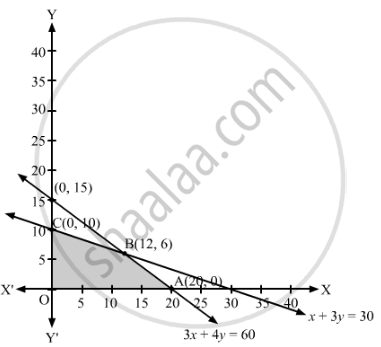# A Manufacturing Company Makes Two Models a and B of a Product. Each Piece of Model a Requires 9 Labour Hours for Fabricating and 1 Labour Hour for Finishing. - Mathematics

Sum

A manufacturing company makes two models A and B of a product. Each piece of model A requires 9 labour hours for fabricating and 1 labour hour for finishing.  Each piece of model B requires 12 labour hours for fabricating and 3 labour hours for finishing. For fabricating and finishing, the maximum labour hours available are 180 and 30 respectively. The company makes a profit of ₹8000 on each piece of model A and ₹12000 on each piece of model B. How many pieces of model A and model B should be manufactured per week to realise a maximum profit? What is the maximum profit per week?

#### Solution

Suppose x pieces of model A and y pieces of model B are manufactured per week.

Since each piece of model A requires 9 labour hours and each piece of model B requires 12 labour hours for fabricating, therefore, x pieces of model A and y pieces of model B require (9x + 12y) labour hours for fabricating. But, the maximum labour hours available for fabricating are 180.

∴ 9x + 12≤ 180

⇒ 3x + 4≤ 60

Similarly, each piece of model A requires 1 labour hour and each piece of model B requires 3 labour hours for finishing, therefore, x pieces of model A and y pieces of model B require (x + 3y) labour hours for finishing. But, the maximum labour hours available for finishing are 30.

∴ x + 3≤ 30

The profit from each piece of model A is ₹8,000 and from each piece of model B is ₹12,000. Therefore, the total profit from x pieces of model A and y pieces of model B is ₹(8,000+ 12,000y).

Thus, the given linear programming problem is

Maximise Z = 8000+ 12000y

subject to the constraints

3x + 4≤ 60

x + 3≤ 30

x, y ≥ 0

The feasible region determined by the given constraints can be diagrammatically represented as,The coordinates of the corner points of the feasible region are O(0, 0), A(20, 0), B(12, 6) and C(0, 10).

The value of the objective function at these points are given in the following table.

 Corner Point Z = 8000x + 12000y (0, 0) 8000 × 0 + 12000 × 0 = 0 (20, 0) 8000 × 20 + 12000 × 0 = 160000 (12, 6) 8000 × 12 + 12000 × 6 = 168000    → Maximum (0, 10) 8000 × 0 + 12000 × 10 = 120000

The maximum value of Z is 168000 at x = 12, y = 6.

Hence, the manufacturing company should produce 12 pieces of model A and 6 pieces of model B to realise maximum profit. The maximum profit is ₹1,68,000.

Concept: Graphical Method of Solving Linear Programming Problems
Is there an error in this question or solution?

#### APPEARS IN

RD Sharma Class 12 Maths
Chapter 30 Linear programming
Exercise 30.4 | Q 43 | Page 56